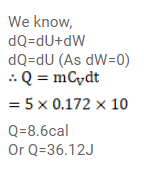Deepak Scored 45->99%ile with Bounce Back Crack Course. You can do it too!

# 5g of a gas is contained in a rigid container and

Question:

$5 \mathrm{~g}$ of a gas is contained in a rigid container and is heated from $15^{\circ} \mathrm{C}$ to $25^{\circ} \mathrm{C} .$ Specific heat capacity of the gas at constant volume is $0.172 \mathrm{cal} / \mathrm{g}-{ }^{\circ} \mathrm{C}$ and the mechanical equivalent of heat is $4.2 \mathrm{~J} / \mathrm{cal}$. Calculate the change in the internal energy of the gas.

Solution: